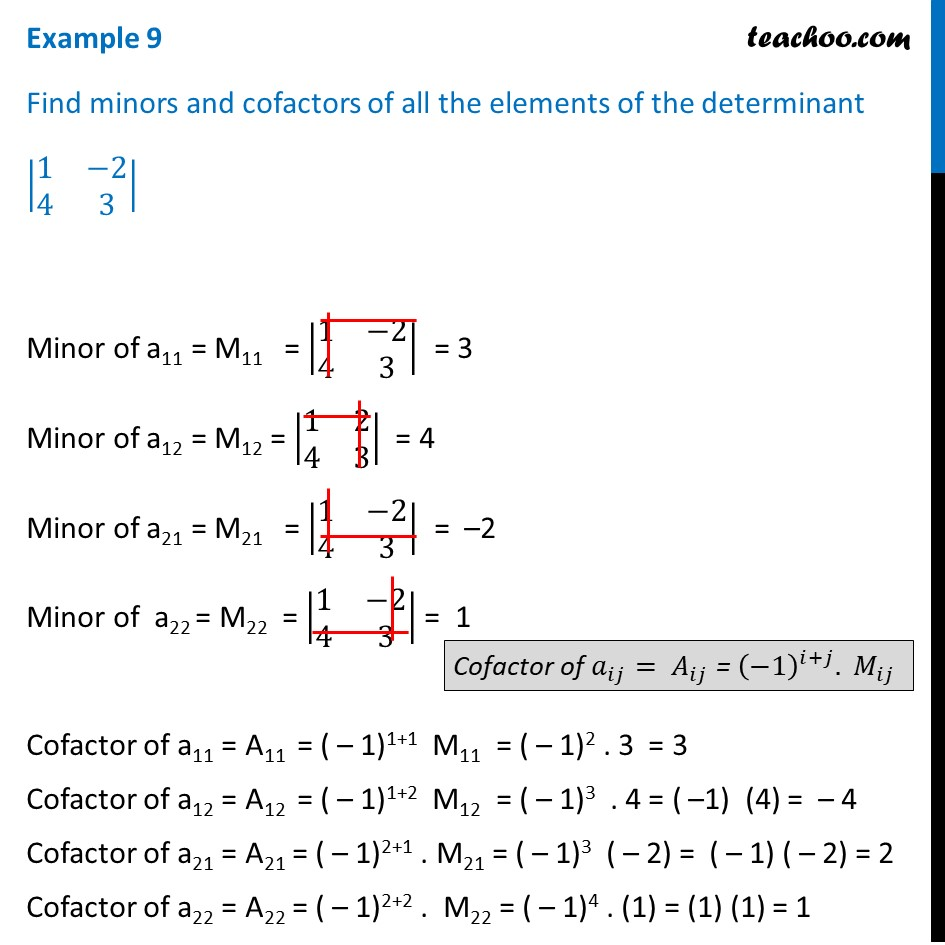Examples

Chapter 4 Class 12 Determinants
Serial order wiseLearn in your speed, with individual attention - Teachoo Maths 1-on-1 Class

### Transcript

Example 9 Find minors and cofactors of all the elements of the determinant |■8(1&−2@4&3)| Minor of a11 = M11 = |■8(1&−2@4&3)| = 3 Minor of a12 = M12 = |■8(1&2@4&3)| = 4 Minor of a21 = M21 = |■8(1&−2@4&3)| = –2 Minor of a22 = M22 = |■8(1&−2@4&3)| = 1 Cofactor of a11 = A11 = ( – 1)1+1 M11 = ( – 1)2 . 3 = 3 Cofactor of a12 = A12 = ( – 1)1+2 M12 = ( – 1)3 . 4 = ( –1) (4) = – 4 Cofactor of a21 = A21 = ( – 1)2+1 . M21 = ( – 1)3 ( – 2) = ( – 1) ( – 2) = 2 Cofactor of a22 = A22 = ( – 1)2+2 . M22 = ( – 1)4 . (1) = (1) (1) = 1